Geometric Sequences and Series
 Home > Lessons > Geometric Sequences and Series Updated February 27th, 2019
Introduction

MATHguide has a video with guided notes to help you understand geometric sequences. Access the guided notes and then proceed with the video. This activity/video addresses two Common Core State Standards: HSF.BF.A.1.A and HSF.BF.A.2.ideo: Geometric Sequencesctivity: Geometric Sequences: Guided Notes

If you rather, you can continue on with this text-based lesson to learn about geometric sequences and series.

Sequences of numbers that follow a pattern of multiplying a fixed number from one term to the next are called geometric sequences. The following sequences are geometric sequences:

Sequence A:   1 , 2 , 4 , 8 , 16 , ...
Sequence B:   0.01 , 0.06 , 0.36 , 2.16 , 12.96 , ...
Sequence C:   16 , -8 , 4 , -2 , 1 , ...

For sequence A, if we multiply by 2 to the first number we will get the second number. This works for any pair of consecutive numbers. The second number times 2 is the third number: 2 × 2 = 4, and so on.For sequence B, if we multiply by 6 to the first number we will get the second number. This also works for any pair of consecutive numbers. The third number times 6 is the fourth number: 0.36 × 6 = 2.16, which will work throughout the entire sequence.

Sequence C is a little different because it seems that we are dividing; yet to stay consistent with the theme of geometric sequences, we must think in terms of multiplication. We need to multiply by -1/2 to the first number to get the second number. This too works for any pair of consecutive numbers. The fourth number times -1/2 is the fifth number: -2 × -1/2 = 1.

Because these sequences behave according to this simple rule of multiplying a constant number to one term to get to another, they are called geometric sequences. So that we can examine these sequences to greater depth, we must know that the fixed numbers that bind each sequence together are called the common ratios. Mathematicians use the letter r when referring to these types of sequences.

Mathematicians also refer to generic sequences using the letter a along with subscripts that correspond to the term numbers as follows:

Generic Sequence: a1, a2, a3, a4, ...

This means that if we refer to the tenth term of a certain sequence, we will label it a10. a14 is the 14th term. This notation is necessary for calculating nth terms, or an, of sequences.

The r-value can be calculated by dividing any two consecutive terms in a geometric sequence. The formula for calculating r is...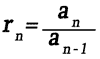...where n is any positive integer greater than 1.

In order for us to know how to obtain terms that are far down these lists of numbers, we need to develop a formula that can be used to calculate these terms. If we were to try and find the 20th term, or worse the 2000th term, it would take a long time if we were to simply multiply a number -- one at a time -- to find our terms.

If we had to find the 400th term of sequence A above, we would undertake a tedious task had we decided to multiply by two each step of the way all the way to the 400th term. Luckily, there is a way to arrive at the 400th term without the need for calculating terms 1 through 399.

The formula for the general term for each geometric sequence is...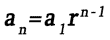1. Let's examine sequence A so that we can find a formula to express its nth term.

If we match each term with it's corresponding term number, we get:

 n 1 2 3 4 5 . . . Term 1 2 4 8 16 . . .

The fixed number, called the common ratio (r), is 2; so, the formula will be an = a12n - 1 or an = (1)2n - 1 or...

an = 2n - 1

For sequence A above, the rule an = 2n - 1 would give the values...
21 - 1 = 20 = 1
22 - 1 = 21 = 2
23 - 1 = 22 = 4
24 - 1 = 23 = 8
25 - 1 = 24 = 16

Now if we were asked to find the 12th term in this sequence, we would calculate like so:

a12 or 212 - 1 which is equal to 211 = 2048.

So, a11 = 2048, or the 11th term is 2048.

Likewise, the 20th term would be a20 = 219 = 524288.

2. Let's take a look at sequence B.

 n 1 2 3 4 5 . . . Term 0.01 0.06 0.36 2.16 12.96 . . .

The fixed number, r, is 6. So the formula will be...

an = (0.01)6n - 1
For the sequence above, the rule an = (0.01)6n - 1 would give the values...

(0.01)61 - 1 = (0.01)60 = (0.01)(1) = 0.01
(0.01)62 - 1 = (0.01)61 = (0.01)(6) = 0.06
(0.01)63 - 1 = (0.01)62 = (0.01)(36) = 0.36
(0.01)64 - 1 = (0.01)63 = (0.01)(216) = 2.16
(0.01)65 - 1 = (0.01)64 = (0.01)(1296) = 12.96

If we wanted to calculate the 8th term, we would calculate like so...

a8 = (0.01)68 - 1 = (0.01)67 = (0.01)(279936) = 2799.36.

If we needed the 12th term, we would calculate like so:

a12 = (0.01)612 - 1 = (0.01)611 = (0.01)(362797056) = 3627970.56. As one can see, the general formula is very handy when n is large.

3. Now let's do the third and final example with sequence C....

 n 1 2 3 4 5 . . . Term 16 -8 4 -2 1 . . .

The common ratio is -1/2. So the formula will be ...

an = (16)(-1/2)n - 1
For sequence C, the rule an = (16)(-1/2)n - 1 would give the values...

(16)(-1/2)1 - 1 = (16)(-1/2)0 = (16)(1) = 16
(16)(-1/2)2 - 1 = (16)(-1/2)1 = (16)(-1/2) = -8
(16)(-1/2)3 - 1 = (16)(-1/2)2 = (16)(1/4) = 4
(16)(-1/2)4 - 1 = (16)(-1/2)3 = (16)(-1/8) = -2
(16)(-1/2)5 - 1 = (16)(-1/2)4 = (16)(1/16) = 1

If for some reason we needed the 11th term, we would calculate like so...

a11 = (16)(-1/2)11 - 1 = (16)(-1/2)10 (16)(0.0009765625) = 0.015625.

Similarly, a16 is equal to...

a16 = (16)(-1/2)16 - 1
a16 = (16)(-1/2)15
a16 = (16)(-0.000030517578125)
a16 = -0.00048828125uizmaster: Finding Formula for General Term

It may be necessary to calculate the number of terms in a certain geometric sequence. To do so, we would need to know two things.

We would need to know a few terms so that we could calculate the common ratio and ultimately the formula for the general term. We would also need to know the last number in the sequence.

Once we know the formula for the general term in a sequence and the last term, the procedure is relatively uncomplicated, but the technique needed to complete the procedure is complicated.

Set the last term equal to the formula for the general term. Since the formula uses the variable n to calculate terms, we can also use it to determine the term number for any given term.

If we again look at sequence A above, let's use the formula that was found to calculate term values, an = a1rn - 1. If we knew that 256 was a number in the sequence (1, 2, 4, 8, 16, ..., 256 ) we would set the number 256 equal to the formula an = a1rn - 1 and get 256 = 2n - 1. Solving this equation using proper techniques requires the use of logarithms and would yield n = 9. [We could use the method of guessing and checking to arrive at the same value.] This means that there are 9 terms in the mentioned sequence and that the 9th term, a9, is equal to 256.

Let's look at a portion of sequence C. If the sequence went from 16 to -1/8, we would have: 16, -8, 4, ..., -1/8. We would use the formula for the general term...

an = (16)(-1/2)n - 1

...and set it equal to the last term, -1/8. We would get -1/8 = (16)(-1/2)n - 1. Solving this equation [with the use of either logarithms or the method of guessing and checking] allows us to arrive at n = 8. This means that there are 8 terms in the sequence and that a8 = -1/8.

Given our generic arithmetic sequence a1, a2, a3, a4, ..., an, we can look at it as a series: a1 + a2 + a3 + a4 + ... + an. As we can see, the only difference between a sequence and a series is that a sequence is a list of numbers and a series is a sum of numbers.

There exists a formula that can add a finite list of these numbers and a formula for an infinite list of these numbers. Here are the formulas...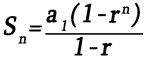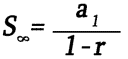...where Sn is the sum of the first n numbers, a1 is the first number in the sequence, r is the common ratio of the sequence, and -1 < r < 1 for infinite series. Let's use examples to investigate both formulas.

Formula One: Finite Sum

1.     If we wanted to add the first 7 terms of sequence A, we would know that a1 = 1, r = 2 and n = 7. We would then plug those numbers into the formula and get:So, S7 = 127.

2.     If we wanted to add the first 10 terms of sequence B, we would know that a1 = 0.01, r = 6 and n = 10. We would then plug those numbers into the formula and get:So, S10 = 120932.35.ideo: Sum of a Finite Geometric Seriesuizmaster: Finding the Sum of a Finite Series

Formula Two: Infinite Sum

1.     If we had to add an infinite number of terms of sequence A, we would first see if it met the requirement of the formula for the common ratio, r. If r is between -1 and 1, an infinite term sum exists. Since sequence A has an r-value of 2 and 2 falls outside the acceptable range, there is no finite sum to that infinite list of numbers.

2.     Let's take an infinite number of terms from sequence C. If we had 16 + (-8) + 4 + (-2) + 1 + ..., then we would know that a1 = 16 and r = -1/2. Since r lies within the acceptable limits, a finite sum exists. Using the infinite term sum formula, we get: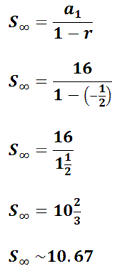3.     If we had to add the infinite sum 27 + 18 + 12 + 8 + ..., we would first identify the first term a1 and the common ratio r, which are 27 and 2/3 respectively. Since r lies between 1 and -1, the formula will work and a finite sum exists for the infinite series. The formula yields: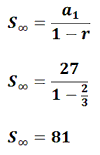ideo: Sum of an Infinite Geometric Seriesuizmaster: Finding the Sum of an Infinite Series

 Instructional Videos Here are the available videos for this content:ideo: Geometric Sequencesideo: Sum of a Finite Geometric Seriesideo: Sum of an Infinite Geometric Series Interactive Quizmasters After reading the lesson, try our quizmaster. MATHguide has developed numerous testing and checking programs to solidify skills demonstrated in this lesson. The following quizmasters are available:uizmaster: Finding Formula for General Termuizmaster: Finding the Sum of a Finite Seriesuizmaster: Finding the Sum of an Infinite Series Related Lessons and Quizmasters After reading the lesson, try a related lesson.esson: Arithmetic Sequences and Seriesesson: Logarithmsesson: Algebra Magicesson: Sigma Notation: Geometric Seriesuiz: Determine the Functionuiz: Find the Pattern